+
Entity-Relationship Model
Relational Database Model
0
of 0 possible points

# Entity-Relationship Model

##### Rating:
(0)
Author: Sophia Tutorial
##### Description:

Recall the notations for Chen, Crow's Foot and class diagram.

(more)
Tutorial

what's covered
This tutorial explores the entity-relationship diagram with the Chen and Crow’s Foot notation as well as the class diagram in two parts:
1. Introduction
2. Notations

## 1. Introduction

The entity-relationship model was created to help describe the relationships in a graphical format. You will hear the term of an entity-relationship diagram (ERD) or an entity-relationship model (ERM) quite often to describe the entities and the relationships that they model.

Peter Chen was the first to introduce the entity-relationship data model in 1976. The ER model had entities or tables that were represented in the ERD by a rectangle which is also known as an entity box. The name of the entity or tale is written in the center of the rectangle. The entity name would generally be written in capital letters and in the singular form.

The entity is mapped to a relational table. An entity can consist of a set of attributes that has characteristics of the entity. For example, the ARTIST entity would have the artist_id and the artist_name. Then we would have relationships described the associations between the entities. The data relationships would either one-to-many, many-to-many, or one-to-one. These relationships show the connectivity between those entities. Generally, the name of the relationship would be a verb that explains what the relationship is. In our example, an ARTIST can RECORD many ALBUMs.

There are different notations that are used to do so but there are three common ones including Chen notation, Crow’s Foot, and the class diagram notation that we will explore further. Chen notation is used to help illustrate modeling concepts and a great notation to use while performing the initial design. However, it can get messy fairly quickly as more relationships, entities, and attributes are added. Crow’s foot notation is the most widely used standard. UML class diagram notation is used for object-oriented or object/relational databases.

## 2. Notations

The Chen notation is based on Peter Chen’s approach. For a relationship, we will have each entity represented by the rectangle and connected by a diamond that shows the relationship name within the diamond. On the relationship line, you will see the representation of the connectivity to see if it is a one-to-one relationship, a one-to-many relationship, or a many-to-many relationship. Attributes of an entity are listed in ovals connected to the table.

Chen notation for a one-to-one relationship would look like the following between the table1 and table2: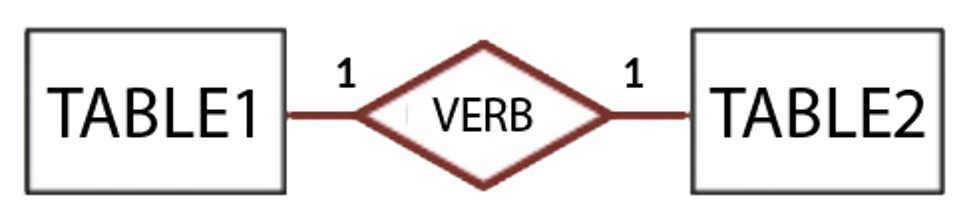Chen notation for a one-to-many relationship would look like the following between the table1 and table2: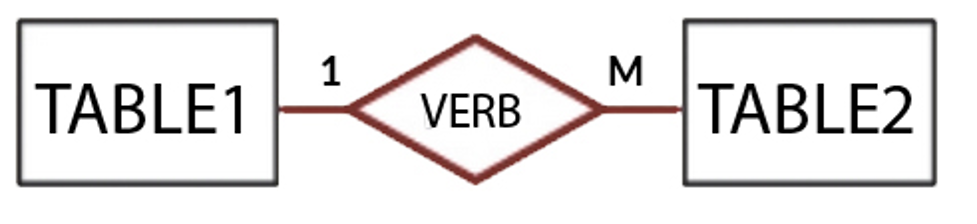Chen notation for a many-to-many relationship would look like the following between the table1 and table2: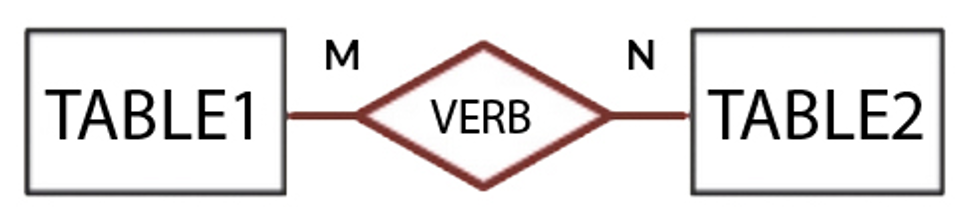The Crow’s foot notation is slightly different where the relationship displays the relationship name above the relationship line instead of in a diamond. The rectangles contain the table name at the top and then list the attributes below the table name one after another. The relationship shows a short line segment if it is on the “one” side and a crow’s foot if it is on the "many" side.

Crow’s foot notation for a one-to-one relationship would look like the following between the table1 and table2: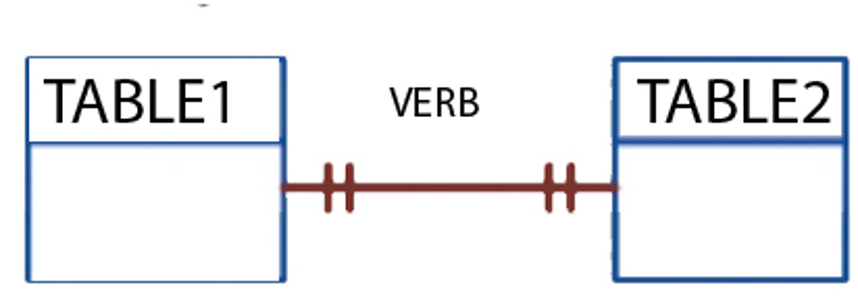Crow’s foot notation for a one-to-many relationship would look like the following between the table1 and table2: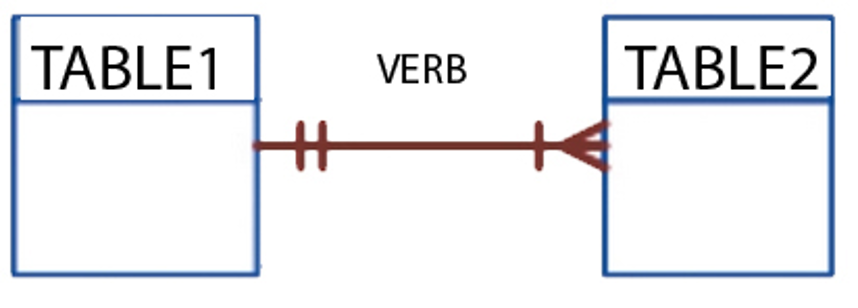Crow’s foot notation for a many-to-many relationship would look like the following between the table1 and table2: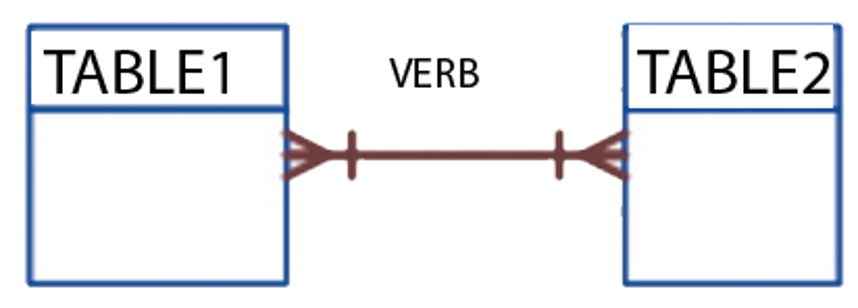In a class diagram, it uses UML notation that is similar to the crow’s foot notation where the class/entity name is at the top following by the attributes in the rectangle below it and then methods/functionality below that. The connectivity is defined with symbols on each side like 1..1 for one and 1..* for many.

UML class diagram notation for a one-to-one relationship would look like the following between the table1 and table2: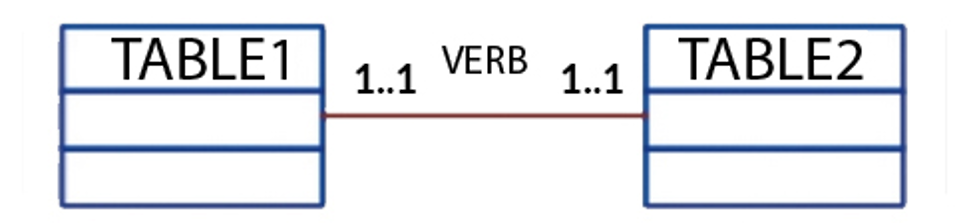UML class diagram notation for a one-to-many relationship would look like the following between the table1 and table2: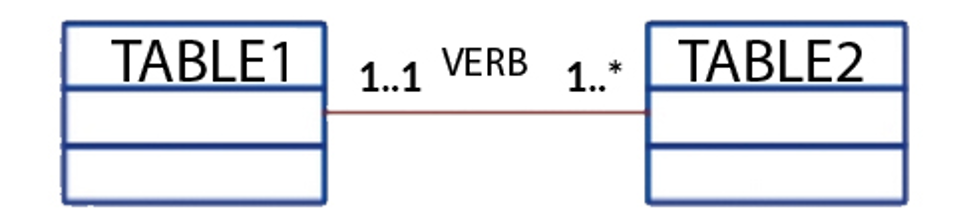UML class diagram notation for a many-to-many relationship would look like the following between the table1 and table2: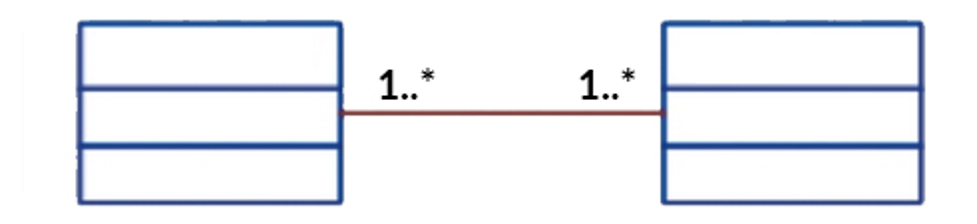summary
There are three main notations used to describe entity relationship diagrams with Chen’s notation, Crow’s Foot notation, and UML Class Diagram notation.

Source: Authored by Vincent Tran

Rating Header